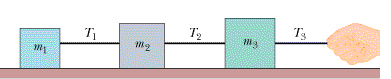Ts121119T,in

Question

In the figure, three connected blocks are pulled to the right on a horizontal frictionless table by a force of magnitude T3 = 78.7 N. If m1 = 15.7 kg, m2 = 27.0 kg, and m3 = 39.9 kg, calculate the tension T2.help_outlineImage TranscriptioncloseTs 12 1119 T, in fullscreen
Step 1

Free body diagram for all the three blocks is as shown below:

Step 2

Since there no motion taking place along vertical direction, the normal force N and the mg force will get neutralized for all the three blocks.

N = mg

Step 3

Since the resultant force along horizontal direction is not zero...

Want to see the full answer?

See Solution

Want to see this answer and more?

Our solutions are written by experts, many with advanced degrees, and available 24/7

See Solution
Tagged in

Newtons Laws of Motion